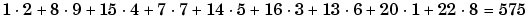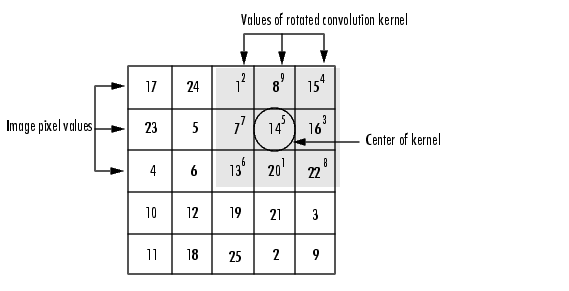Image Processing Toolbox User's GuideConvolution

Linear filtering of an image is accomplished through an operation called convolution. Convolution is a neighborhood operation in which each output pixel is the weighted sum of neighboring input pixels. The matrix of weights is called the convolution kernel, also known as the filter. A convolution kernel is a correlation kernel that has been rotated 180 degrees.

For example, suppose the image is

• ```A = [17  24   1   8  15
23   5   7  14  16
4   6  13  20  22
10  12  19  21   3
11  18  25   2   9]
```

and the convolution kernel is

• ```h = [8   1   6
3   5   7
4   9   2]
```

The following figure shows how to compute the (2,4) output pixel using these steps:

1. Rotate the convolution kernel 180 degrees about its center element.
2. Slide the center element of the convolution kernel so that it lies on top of the (2,4) element of `A`.
3. Multiply each weight in the rotated convolution kernel by the pixel of `A` underneath.
4. Sum the individual products from step 3.

Hence the (2,4) output pixel isComputing the (2,4) Output of ConvolutionLinear Filtering Correlation